# Some Questions About Trinions

(diff) ← Older revision | Latest revision (diff) | Newer revision → (diff)
Jump to: navigation, search

This paperlet was born as 06-1350/Class Notes for Tuesday October 10.

## The Questions

Question 1. Can you embed a trinion (a.k.a. a sphere with three holes, a pair of pants, or a band theta graph) in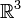${\mathbb R}^3$ so that each boundary component would be unknotted yet each pair of boundary components would be knotted? How about, so that at least one pair of boundary components would be knotted?

Dror's Speculation. Yes and yes.

Question 2. A trinion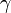$\gamma$ is embedded in${\mathbb R}^3$ so that its boundary is the trivial 3-component link. Does it follow that$\gamma$ is trivial?

Dror's Speculation. No.

Question 3. Suppose two trinions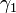$\gamma_1$ and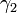$\gamma_2$ are knotted so that the pushforwards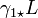$\gamma_{1\star}L$ and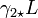$\gamma_{2\star}L$ are equal for any link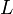$L$ which is "drawn" on the parameter space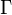$\Gamma$ of$\gamma_1$ and$\gamma_2$. Does it follow that$\gamma_1$ and$\gamma_2$ are equivalent?

Dror's Speculation. I'm clueless.

Question 4. A trinion$\gamma$ is embedded in${\mathbb R}^3$ so that its "strapped boundary" is equivalent to the strapped boundary of the trivially embedded trinion. Does it follow that$\gamma$ is trivial?

Dror's Speculation. If yes, it will have terrific consequences. If no, it will explain some of the misery we encounter when we deal with "associators". I would really like to understand this one.

Of course, these questions all can and should be generalized to arbitrary knotted graphs.

## Why do I care?

In the paperlet Algebraic Knot Theory - A Call for Action I argue that knotted graphs are very valuable. The underlying thread in the questions above is "Can a knotted graph be read from the knots drawn on it"? If yes, it may be that knotted graphs are not needed after all, though perhaps knot theory should be studied with more attention given to the likes of the strapped trinion above. Somehow "yes" seems like the less likely answer to me, though I'd like to see a definitive "no".